We use cookies and other tracking technologies to improve your browsing experience on our site, analyze site traffic, and understand where our audience is coming from. To find out more, please read our privacy policy.

By choosing 'I Accept', you consent to our use of cookies and other tracking technologies.

We use cookies and other tracking technologies to improve your browsing experience on our site, analyze site traffic, and understand where our audience is coming from. To find out more, please read our privacy policy.

By choosing 'I Accept', you consent to our use of cookies and other tracking technologies. Less

We use cookies and other tracking technologies... More

# Login or registerto boost this post!

Show some love to the author of this blog by giving their post some rocket fuel 🚀.

# Login or register to start working on this issue!

Engineers who find a new job through Functional Works average a 15% increase in salary 🚀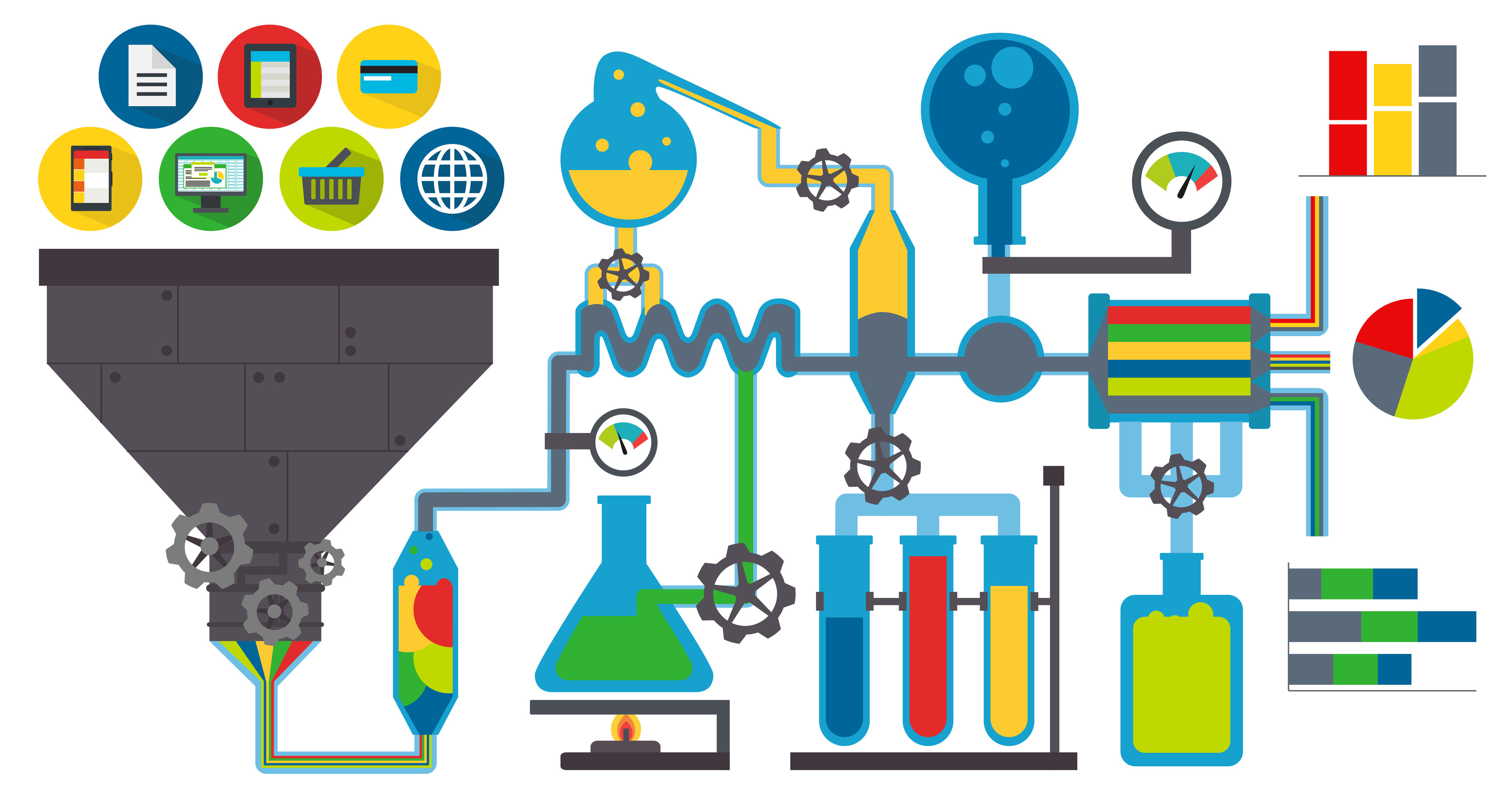# Recognising Handwritten Digits with Scala

Nejc Ilenic 29 June, 2018 | 11 min readIn the past few months I’ve started writing Scala code extensively and for someone who’s migrated from Python, I have to admit that operating in a statically typed setting feels way safer than I was willing to acknowledge before.

The main reason for my Python journey was its machine learning ecosystem and as soon as I started looking into the state of ML in Scala, I realised that there is a huge gap between the two languages in terms of available libraries. Particularly, I was looking for a Scala alternative to what scikit-learn has to offer in Python and it’s fair to say I felt discouraged having found out that there isn’t one. My disappointment quickly turned into eagerness when I remembered that I could start building it myself, and so I did.

The purpose of this post is twofold: I want to spread the word about doddle-model and present to the non-ML audience how the problem of recognising handwritten digits might be approached, in a highly intuitive, rather than technical, manner.

## Introduction

The library is called doddle-model and the name is trying to emphasise its ease of use.

doddle British, informal: a very easy task, e.g. “This printer’s a doddle to set up and use.”

It is essentially an in-memory machine learning library that can be summed up with three main characteristics:

• it’s built on top of Breeze
• it provides immutable objects that are a doddle to use in parallel code
• it exposes its functionality through a scikit-learn-like API

It should also be noted that the project is in the early-stages of development and any kind of contributions are much appreciated.

## Problem Definition

Suppose your grandma asks you to help her digitise an old handwritten telephone book by saving the contacts on a mobile phone. Unfortunately, the list is quite long and as a savvy engineer, you quickly realise that an important task like this must be automated. You also remember hearing of a popular database of labeled handwritten digits containing 70.000 images and their corresponding labels, that you might be able to use to help your grandma out. You can take a look at Image 2 for some of the examples.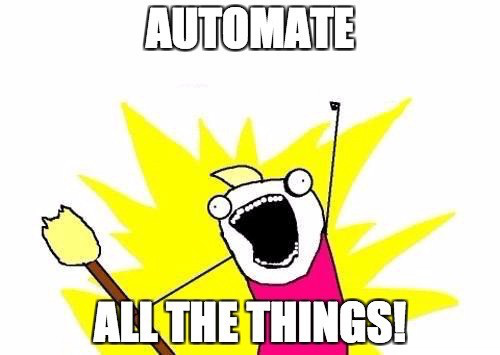We’re going to assume that we’ve found a way to scan the telephone book and convert each telephone number into a set of 28x28 grayscale images, where each image represents a single digit. Additionally, we’ll ignore the fact that we don’t know how to digitise handwritten letters and will thus only focus on numbers themselves.

Given the current scenario, we are interested in devising a function that takes a vector of 784 integers between 0 and 255 as an input and produces a single integer between 0 and 9 (our digit) at the output. Remember that grayscale images only contain intensity information, hence a single integer for each of the 28x28 pixels (we simply flatten the 28x28 matrix into a single vector to obtain 784 values).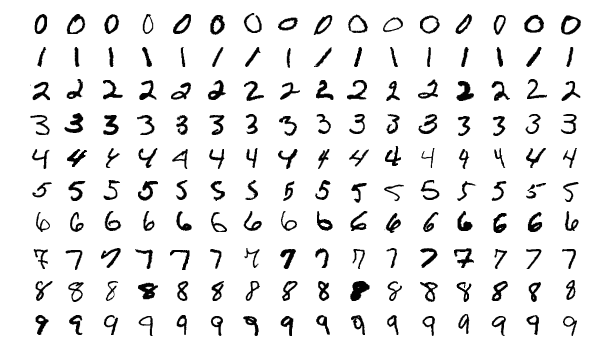## The First Approach

We’ll denote the input pixel vector by x and the output scalar by y. The function we are looking for is then f(x) = y. To make things convenient we’ll model the output as a categorical variable, and this is nothing more than saying that its values fall into any one of a set of unordered categories, i.e. one of {0, 1, 2, 3, 4, 5, 6, 7, 8, 9} in our case. If you take a look at the Categorical distribution you can see that it’s parametrised by a probability vector where each of the elements represents a probability for the k-th category and that they all sum to 1 since no other categories exist. We can incorporate this into our function, by saying that the output of f is not a single scalar y, but a vector yProb with 10 elements, where each element represents a probability for each of the possible digits. Our function then becomes f(x) = yProb.

## Our Function Definition

A possible approach to obtain a real-valued scalar from vector x is to simply calculate a linear combination of the values in x. Let’s see how we could write this down. Remember that x consists of 784 integers, namely pixel1, pixel2, …, pixel783, pixel784, so to calculate a linear combination of these, we need 785 weights wi to obtain z = w0 + w1pixel1 + w2pixel2 + … + w783pixel783 + w784pixel784. If we insert an element with value 1 at the beginning of x, we can write this down as a dot product of x and w (all weights w_i in a single vector), obtaining z = xᵀw.

That was easy, but currently, we only have a single score z and we actually need a vector of 10 at the output. Well, the simplest thing we could do is calculate a score 10 times instead of once, each time with a different vector w. We could write this down like so: z0 = xᵀw0, z1 = xᵀw1, …, z10 = xᵀw10. Again, if we concatenate vectors wi in a matrix W (each wi becomes a column in W), we can write this down as a vector-matrix multiplication z = xᵀW.

Let’s take a look at a how our function f currently looks like to recap what we’ve done up until this point. And don’t worry about not knowing what values W holds, we’ll get there in a minute. On the very left side of Image 3 is our flattened image vector x with prepended 1 and it’s multiplied by matrix W to obtain vector z that represents real-valued scores for each of the 10 possible categories (digits). Note that we have a set of weights for every category (each column in W), giving us 10*785 weights in total.There is only one requirement that is missing from our function definition. We specified at the beginning of this section that elements of z have to be in the range [0, 1] and have to sum to 1 in order to be interpreted as probabilities. Well, as it turns out, the [softmax function](https://en.wikipedia.org/wiki/Softmax_function) does exactly what we’re looking for. It’s pretty simple and we won’t go into too much detail here, what we have to know is that it takes our z and returns yProb that may look something like this yProb = [0.024, 0.064, 0.175, 0.475, 0.024, 0.064, 0.175]. If you are interested in the definition of the softmax function follow the provided link.

We’ve finally figured out how our f could be defined! That’s great news, we are almost at the point where we’ll be able to help our grandma with her no-numbers-in-a-mobile-phone problem. The final function definition is the following: f(x) = softmax(xᵀW). It takes a flattened image vector x (well, we mustn’t forget to insert 1 as the very first element) and returns a vector of probabilities for 10 possible digits. Awesome! But wait, we haven’t said anything about the weights W yet?!

## The Weights

Weights W are called model parameters in ML literature and f is called a model. If we initialise W randomly we’ll likely end up with a pretty bad model — the predicted digit (the category with the maximum value from vector yProb ) will be wrong most of the time and we want to find a better approach.

We have one more thing at our disposal: the labeled dataset! Intuitively, we will try to find a set of weights W that works well on the labeled data (we’ll call it training data, and searching for parameters would then be called training) and hope that they also work well on the new data (the scanned digits from our grandma’s phone book). Battle-tested data scientists are probably making faces at this point, since we don’t want to just hope it will do well, we want to measure it and be certain that the model won’t make too many mistakes. We don’t want the model to simply memorise our training examples, but rather to learn about the concept of e.g. digit 2 or digit 9 and to have the ability to recognise new, unseen images of these digits.

Let’s define what works well means in our scenario. What we could do is calculate the proportion of images from the training set that our model got correctly. This metric is called classification accuracy and we can calculate it since we know what the correct labels in the training set are. A naive approach to finding W could then be to simply try random values and return the ones that have the highest accuracy. But sure we can do better than a random search, right?

Again, we don’t want to go into too much detail, but there exists an iterative optimisation algorithm for finding the minimum of a function (take a look at the Wikipedia page of gradient descent for more information). The idea here is that we specify an error function — we measure how bad our model’s predictions are on the training set — and minimise it with respect to parameters W. Gradient descent will then give us some W that works best on our training data in terms of that error function. The concept is visualised in Image 4: the surface is our error function, axes X and Y are two of our values in W (yeah we actually have 10*785 but for the sake of visualisation imagine we only have 2) and the algorithm tries different X’s and Y’s along the red path and finally returns some X and Y for which the value of the function is the lowest. This value is called a function minima and it makes sense in our case since we are looking for a minima of the error function.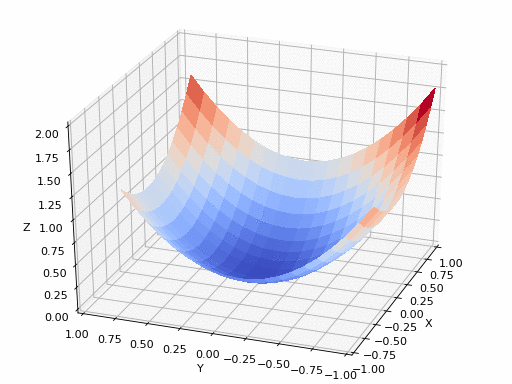Unfortunately, we can’t use something like minus classification accuracy as the error function because gradient descent needs a differentiable function and accuracy isn’t such. The one we can use is called logarithmic loss and it simply says that we calculate the negative logarithm of a probability of the correct category. We know what the correct category is because our training set is labeled, so we simply take the value from yProb that corresponds to that category (let’s call it yProb) and calculate -log(yProb). You can see in Image 5 that the value approaches 0 as yProb gets closer to 1. The final error function is then the sum (or average) of log losses for all examples from our training set. Note that log loss also has other desirable properties that make it a better choice for our error function, but let’s not concern ourselves with details right now.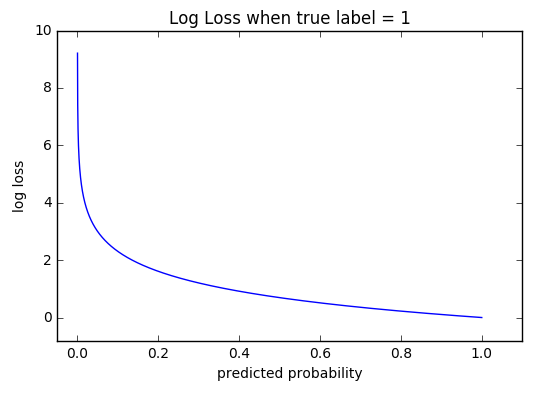And this is actually the essence of our first approach, nothing that couldn’t be implemented in a few lines of Scala! To recap what we’ve done, let‘s take a look at the most important steps again:

• we’ve devised a function f that takes a flattened image vector x as an input and returns a vector yProb with 10 elements, where each element represents a probability for each of the possible digits (parameters W were incorporated into f)
• we’ve used f and a labeled training set to measure how bad our parameters W are by calculating the average log loss over all examples in the training set
• we’ve used an optimisation algorithm that gave us some W that worked best in terms of the log loss error function
• we hope the returned W will also work well for the digits from the telephone book

## The Library

Next, we’ll take a look at how all of the stuff we’ve discussed up to this point can be implemented with a few lines of Scala by using the doddle-model library. To be completely honest, the exact implementation from the library has some important differences with the presented technique that make the whole approach numerically stable and more efficient, but it’s not essential for us to talk about that at this moment. Let’s jump into the code instead.

We’ll use the labeled dataset from mldata.org that consists of 70.000 labeled images. We’ll simulate training and testing data by only using 60.000 images for training and the remaining 10.000 for evaluating performance.

``````val data = loadCsvDataset("mnist.csv", headerLine = false)

// we divide all pixels by 255, putting them in range [0, 1]
// this doesn't change much, but makes optimisation converge faster
val (xTrain, yTrain) = (data(0 until 60000, 0 to -2) / 255.0, data(0 until 60000, -1))
val (xTest, yTest) = (data(60000 to -1, 0 to -2) / 255.0, data(60000 to -1, -1))
``````

If we inspect the shapes, we could see that rows in `xTrain` and `xTest` represent our flattened image vectors (each row is a single image vector) and columns represent the pixel values. `yTrain` and `yTest` hold the actual labels, e.g. the first 10 training images have digits 1, 9, 2, 2, 7, 1, 8, 3, 3 and 7 on them.

``````println(s"Training dataset shape: \${xTrain.rows} rows and \${xTrain.cols} columns")
// Training dataset shape: 60000 rows and 784 columns

println(s"Test dataset shape: \${xTest.rows} rows and \${xTest.cols} columns")
// Test dataset shape: 10000 rows and 784 columns

// let's take a look at some of the first training digits
println(yTrain(0 until 10))
// DenseVector(1.0, 9.0, 2.0, 2.0, 7.0, 1.0, 8.0, 3.0, 3.0, 7.0)
``````

Training the model is then as simple as:

``````import com.picnicml.doddlemodel.linear.SoftmaxClassifier

val softmax = SoftmaxClassifier()

// note that we can use the whole matrix (multiple images) as an input in contrast
// to our definition above where f could only take a single image
val trainedSoftmax = softmax.fit(xTrain, yTrain)
``````

And making predictions looks like:

``````val yTestPred = trainedSoftmax.predict(xTest)
``````

Now that we have model predictions `yTestPred` and also the true labels `yTest` we know are correct, we could check whether the predictions from the model make any sense at all. Let’s first take a look at the first 10 digits from test data and our predictions for them.

``````println(s"First 10 digits from the unseen data:\n\${yTest(0 until 9)}")
// First 10 digits from the unseen data:
// DenseVector(4.0, 3.0, 6.0, 8.0, 6.0, 2.0, 4.0, 4.0, 3.0)

println(s"First 10 predictions for the same images:\n\${yTestPred(0 until 9)}")
// First 10 predictions for the same images:
// DenseVector(4.0, 3.0, 6.0, 8.0, 6.0, 2.0, 4.0, 4.0, 3.0)
``````

That looks like it could work! Let’s calculate classification accuracy on the whole test dataset:

``````import com.picnicml.doddlemodel.metrics.accuracy

println(s"Classification accuracy on the unseen data: \${accuracy(yTest, yTestPred)}")
// Classification accuracy on the unseen data: 0.9222
``````

Not bad! We were able to correctly classify 92.22% of the 10.000 images that were not used during training. That still leaves our grandma with incorrect numbers in her mobile phone, but it’s a good starting point that we can gradually improve!

## Performance

The library is developed with performance in mind. See the doddle-benchmark repository where some side-by-side performance comparisons with scikit-learn are presented.

Breeze (the underlying numerical processing library) utilises netlib-java for accessing hardware optimised linear algebra libraries and this is the main reason for fast execution. For more details see the performance section of the README.

## Conclusion

If you’ve made it up to this point, give yourself a pat on the back, as you know quite a bit about the softmax classifier and how to train it. If you are interested in more there are lots of good courses and resources available for free on the web.

If you are interested in learning more about doddle-model, take a look at the doddle-model-examples repository. It contains code examples for everything that is currently implemented and should serve as a good starting point.

If you have any questions, suggestions, comments or would just like to chat, you can give me a shout on Gitter or on Twitter.

Originally published on towardsdatascience.com